# Rank data Based on the Number of Occurrences

This post will guide you how to rank data by the number of occurrences in excel. How do I rand cells by the number of occurrences with formula in excel.

## Rand Data by Occurrences

Assuming that you have a list of data in your worksheet, and you want to rank those data based on the number of occurrence of those value in the specified range of cells. You need to get the number of occurrences for the selected range of cells, then rank those number using the RANK function. Just do the following steps:

#1 you need to copy the data list firstly and then paste the data into another new column (C1:C8).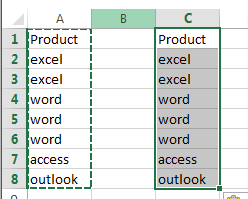#2 go to DATA tab, click Remove Duplicates command under Data Tools group.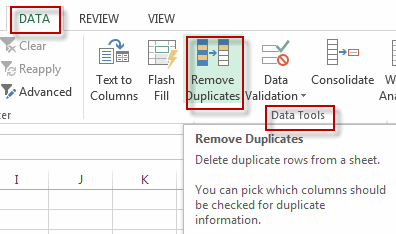#3 click OK button. All duplicate values are removed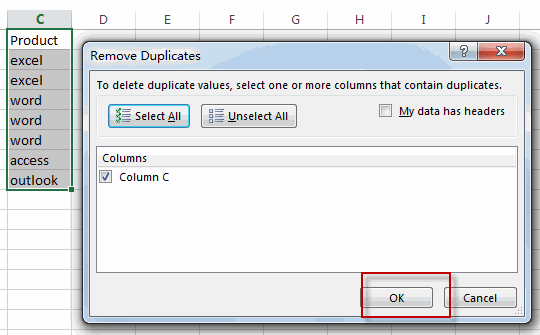#4 type this formula into the formula box of cell D2 to get the occurrence number in the range of cells A1:A8, then press enter key, and drag the AutoFill Handler over other cells to apply this formula.

=COUNTIF(\$A\$1:\$A\$8,C2)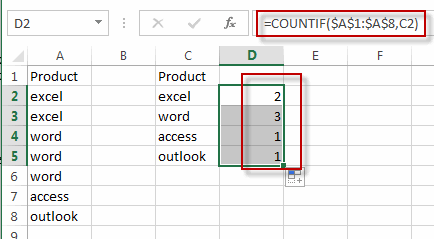#5 type the RANK formula into the formula box of cell E1 to rank the data based on the occurrence number, then press enter key, and drag AutoFill Handler over other cell.

`=RANK(D2,\$D\$2:\$D\$5)`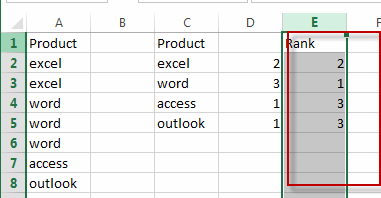### Related Functions

• Excel COUNTIF function
The Excel COUNTIF function will count the number of cells in a range that meet a given criteria. This function can be used to count the different kinds of cells with number, date, text values, blank, non-blanks, or containing specific characters.etc.= COUNTIF (range, criteria)…
• Excel Rank function
The Excel RANK function returns the rank of a given number in a supplied range of cells.  If there are duplicated values in a list of values, it will be set the same rank.The syntax of the RANK function is as below:= RANK (number,ref,[order])….